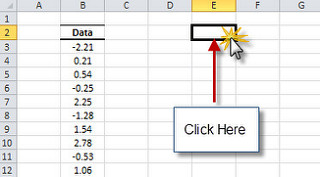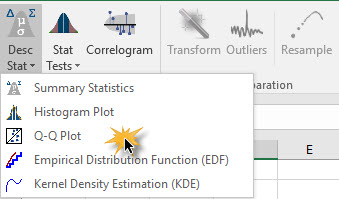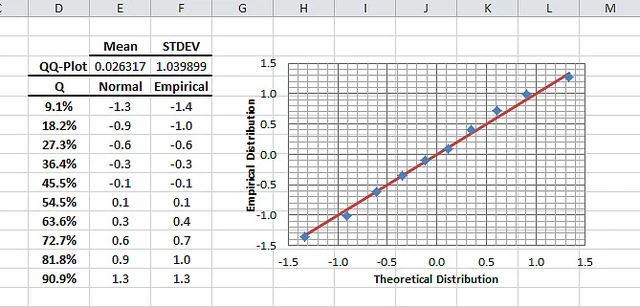# Quantile-Quantile (QQ) Plot

In statistics, a Q-Q Plot (“Q” stands for Quantile) creates a graphical comparison between two distributions by plotting their quantiles against each other.

## Process

NumXL provides an intuitive interface to help Excel users construct a Q-Q Plot of an empirical sample data distribution against a theoretical Gaussian distribution. In this tutorial, we’ll demonstrate the steps to construct a Q-Q Plot using NumXL functions in Excel.

1. Select an empty cell to store the histogram table2. Locate the Descriptive Statistics (DESC STAT) icon in the toolbar (or menu in Excel 2003) and click the down-arrow. When the drop-down menu appears, select “Q-Q Plot”3. The Q-Q Plot dialog box appears
4. Select the cell-range for the input dataNote: The table output range is set to the selected empty cell in your worksheet.
5. Next, select the number of quantiles (number of points in the Q-Q Plot). The number of bins is set initially to 10.
6. Click OK.

## Output

The QQ-Plot wizard generates the output table and plots the graph on the right